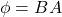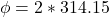## A circular loop of wire with radius 10.0 cm is located in the xy-plane in a region of uniform magnetic field. A field of 2 T is directed in

Question

A circular loop of wire with radius 10.0 cm is located in the xy-plane in a region of uniform magnetic field. A field of 2 T is directed in the z-direction, which is upward. (a) What is the magnetic flux through the loop

in progress 0
2 months 2021-07-31T08:19:11+00:00 1 Answers 1 views 0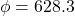Explanation:

From the question we are told that

Radius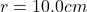Magnetic field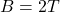Generally the equation for area of circular path is mathematically given by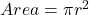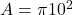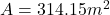Generally the equation for Magnetic flux is mathematically given by